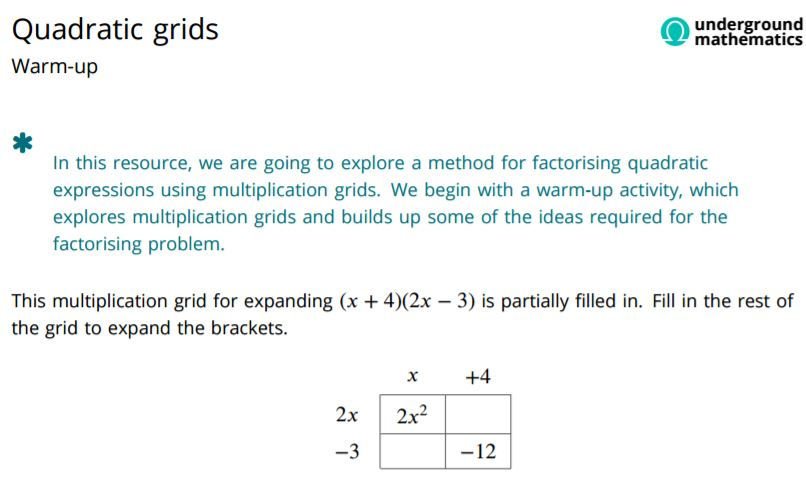When factorising quadratic expressions I always encourage students to check coefficients first, if the coefficient of x2 and the constant are prime for example they clearly do not need elaborate methods.

Some students have difficulty with the splitting the middle term method; if one must have a recipe to follow – try the box method.

Working on Quadratic Grids from Underground Mathematics will help students develop and understand the method.We could illustrate on the brilliant Area Model Algebra from PhET Sims.

For instructions on the method:

Quadratic Factorisation Box method (pdf file)

Perhaps even simpler is Lyszkowski’s method which avoids the manipulation required by conventional methods.

I came across the method through Colin Foster’s wonderful Mathematical Etudes site. To quote Colin Foster:

Mathematical Etudes are creative, imaginative and thought-provoking ways to help learners of mathematics develop their fluency in important mathematical procedures. They are an alternative to traditional, tedious exercises.

Note the Etudes by topic at the foot of the page; Number, Algebra, Geometry, Probability & Statistics are available. Looking at Algebra for example, under Solving Equations we see Connected Quadratics which is where I found Lyszkowski’s method of factorising quadratics.

Comparing the two methods with an example:We could have a look at the general case for the box method :and for Lyszkowski’s method:Try this excellent lesson plan of  Colin Foster’s on Quadratic Equations. I really like the starting activity in this lesson which should promote a deeper understanding of factorising quadratic expressions.